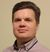# QlikView App Dev

Discussion Board for collaboration related to QlikView App Development.

Announcements
On May 18th at 10AM EDT we will answer your QlikView questions live. REGISTER
cancel
Showing results for
Did you mean:Not applicable

## someone can tell me what this code means ?

Hi All,

I found this calculation ,

and I am trying to understand how the sum() calculate if there is '=' sign  in it .

this is the code :

sum({<DateNum={\$(vCurrentDate)}, [Source Type] = [Source Type] * {"=[Source Type]=3"} \$1 >} [Operational Amount])

what does it means? how is the calculation accrue ?

thanks

Moni

1 Solution

Accepted SolutionsMVP

I believe it's replacing \$1 with ', _YearPeriod = {201412}':

sum({<DateNum={\$(vCurrentDate)}, [Source Type] = [Source Type] * {"=[Source Type]=3"} \$1 >} [Operational Amount])

-->

sum({<DateNum={\$(vCurrentDate)}, [Source Type] = [Source Type] * {"=[Source Type]=3"} , _YearPeriod = {201412} >} [Operational Amount])

Then it evaluates the aggregation incl. the set analysis (see my above link for more about set analysis, also the HELP and search the forum).

8 RepliesMVP

The code between {<  and >} is called set analysis:

A Primer on Set AnalysisMVP

I think without \$1

sum({<DateNum={\$(vCurrentDate)}, [Source Type] = [Source Type] * {"=[Source Type]=3"}  >} [Operational Amount])Contributor III

Hi. Does this expression work?Contributor III

DateNum, [Source Type] and [Operational Amount] are fields of data model, which consist from tables.

vCurrentDate is variable.

Sum([Operational Amount]) is ordinary operation of adding all values of field [Operational Amount].

{<DateNum={\$(vCurrentDate)}, [Source Type] = [Source Type] * {"=[Source Type]=3"} \$1 >} is expression of set analysis, which sets selections in fields "DateNum" and [Source Type] for reducing amount of values in field [Operational Amount], which will be add.

DateNum={\$(vCurrentDate) sets selection in field "DateNum", which equal variable "vCurrentDate".

[Source Type] = [Source Type] * {"=[Source Type]=3"} sets selection in field [Source Type], which consist from:

1. current selection in field [Source Type];

2. value of field [Source Type], which equal 3.

But total selection will be as crossing between them. Values, which are in both parts will put in total selection.

Sorry for my english.MVP

The \$1 could be part of a dollar sign expansion with parameter (see also the HELP), are you taking this code from a variable definition?Not applicable
Author

the original value is :

=\$(=Replace(Only({1<_MetricID={'-55'}>} _MetricCalc), '\$1', ', \$(vCurrentFilter)'))

the vCurrentFilter  parameter value is _YearPeriod={201412}

I do not understand what it doesNot applicable
Author

the original value is :

=\$(=Replace(Only({1<_MetricID={'-55'}>} _MetricCalc), '\$1', ', \$(vCurrentFilter)'))

the vCurrentFilter  parameter value is _YearPeriod={201412}

\$1' =   sum({< DateNum={"<=\$(vCurrentDate)"} \$1 >} [Budget Transfers])

I do not understand what it doesMVP

I believe it's replacing \$1 with ', _YearPeriod = {201412}':

sum({<DateNum={\$(vCurrentDate)}, [Source Type] = [Source Type] * {"=[Source Type]=3"} \$1 >} [Operational Amount])

-->

sum({<DateNum={\$(vCurrentDate)}, [Source Type] = [Source Type] * {"=[Source Type]=3"} , _YearPeriod = {201412} >} [Operational Amount])

Then it evaluates the aggregation incl. the set analysis (see my above link for more about set analysis, also the HELP and search the forum).